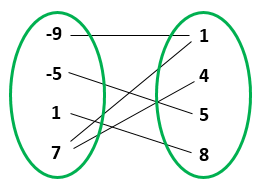Anindya wrote:

> but tbh I don't really have much of a feel for what a relation is, beyond the formal definition.

I tend to visualize relations as matrices of 0's and 1's, or matrices of \$$\texttt{true}\$$'s and \$$\texttt{false}\$$'s. Composing relations is just matrix multiplication using \$$\texttt{and}\$$ and \$$\texttt{or}\$$ instead of \$$\times\$$ and \$$+\$$.

Profunctors are also just glorified matrices, with composition being done by a version of matrix multiplication.

Another way I imagine a relation is this. A set is a bunch of dots. A relation from a set \$$X\$$ to a set \$$Y\$$ is as a bunch of line segments: one joining each dot in \$$X\$$ to each dot in \$$Y\$$ to which its related:Unlike the usual picture of a _function_:you probably don't want to imagine _arrowheads_ on the line segments for a relation! You want to be able to just turn around your mental picture of a relation \$$R \colon X \nrightarrow Y\$$ and see the transpose \$$R^\top \colon Y \nrightarrow X\$$.

Of course each line segment represents a \$$\texttt{true}\$$ in the matrix I mentioned earlier, and each _absence_ of a line segment represents a \$$\texttt{false}\$$. Composing relations has a very simple pictorial interpretation in terms of these line segments... and you can see that it's the same as matrix multiplication using \$$\texttt{and}\$$ and \$$\texttt{or}\$$.

I don't know if this helps, but I feel completely happy with my understanding of relations.... probably because I have a bunch of interlocking ways to think about them... so maybe I can make you happy too.